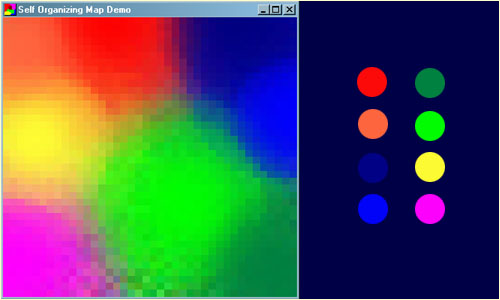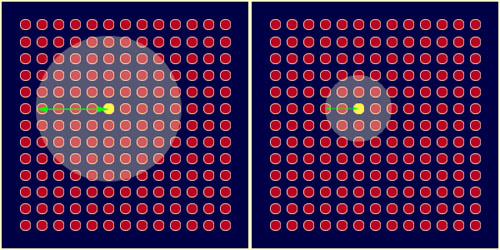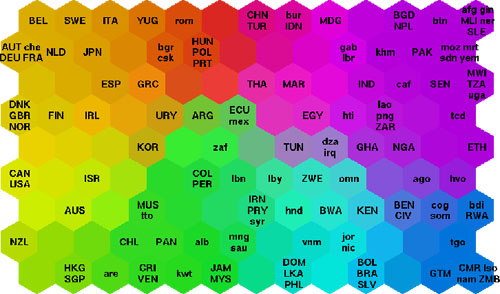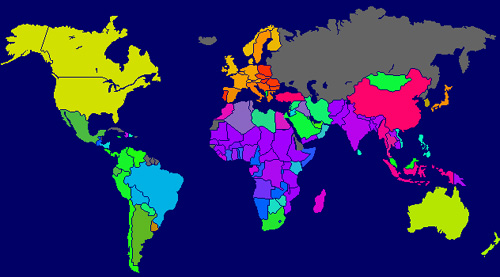# Self Organizing Maps (SOM) in NetLogo

Última modificación: 16 de Diciembre de 2017, y ha tenido 1134 vistas

Etiquetas utilizadas: || || || || ||Self Organizing Maps, or SOM, were created in 1982 by Teuvo Kohonen, professor from the Finland Academy, and provide a way to to represent multidimensional data (vectors) in lower dimensional spaces, usually 2D. In general, different techniques to reduce vectors dimensionality are data compression techniques also known as Vector Quantization. One important fact in Kohonen technique is that we also obtain some conservation of topological properties from the original data.

A tipical example to see SOM working is based in the projection of colors (associated to 3D vectors from, for example, the RGB components) to a 2D space. Next figure show a trained SOM to recognize the 8 colors from the left. In the obtained 2D representation, similar colors are located in adjacent regions.As machine learning algorithm, SOM is in the smaller bag of unsupervised methods, what means we have no feedback about a goal to require, but it provides a distribution of the vectors considering their similarities. From a technical point of view, it considers a network of nodes in a similar way as artificial neural networks.

## Network Architecture

SOM algorithm has a 2 layer network architecture: we have a learning nodes layer, which we mind the geometrical-topological relation between them, and that finally will contain the information about the resulting representation; and we have one more layer with the input nodes, where the original vectors will be feeded to the network during the training process. Also, there exist connections between all the nodes of both layers.

Next figure shows a possible 2D architecture for a SOM training. As in other similar models, the idea of the algorithm is to find correct weights for the connections between to give a correct representation of the input data in the geometrical structure of learning nodes.In fact, as we don't mind the geometrical or topological representation of input nodes, it is usual to work only with the representation of learning nodes, and hence the weights are shown as vectors in these nodes (every element in this vector is the weight of the connection with the correspondent input node). In this way, if the input layer has size $n$ (the dimension of the original space), then every weight vector , $W$, will have dimension $n$.

In our first NetLogo implementation we will make use of patches as training nodes, and we will store in a custom variable the weights of the connections with the 3 input nodes (3D, RGB colors).

patches-own [
weight ]


The training vectors will be stored in a global variable:

; TSet stores the Training Set
globals [
TSet
]


## Learning Algorithm

Roughly, since there is no goal vector to approximate, what we will do is, where the nodes of the of the network have weight vectors coincident with original vector, the surrounding learning nodes tend to approximate their respective weights to the same value. In this way, starting from a initical distribution of weights (usually, a random one), SOM network tends to approximate a stable weights distribution. Every one of this stabilized areas becomes a properties classifier. Once the network stabilizes, any new vector will stimulate the area with similar weights.

The Setup procedure initially assigns a weight to every node. It can be done randomly, or using a grey gradient, by means of the Init chooser control in the interface.

to setup
ca
ifelse (Init = "Random")
[ ; Associate a random vector to every patch
set weight n-values 3 [random-float 1]
set pcolor rgb (255 * item 0 weight) (255 * item 1 weight) (255 * item 2 weight) ]
]
[ ; Associate a gradient grey vector to every patch
set pcolor scale-color black ((pxcor + pycor + 2 * max-pxcor) / (4 * max-pxcor)) 0 1
set weight map [? / 255] extract-rgb pcolor ]
]  ; Create a random list of random RGB-colors
set TSet n-values TSet-size [n-values 3 [random-float 1]]
reset-ticks
end


In a more detailed form, the steps to follow in the training process are:

1. Every node is initialized with a (random) weight. Usually, a vector from $[0,1]^n$,

2. A vector from the input data is selected, $V$.

3. The node with the most similar weight to $V$, we will denote it as Best Matching Unit (BMU). For that, we simply compute the distances between all the weight vectors of all the learning nodes and $V$ (by efficiency reasons, we will not apply the square root, because we only need to know where the minimum is).

4. Compute the radius of the BMU neighbourhood. This radius will start with a big value (to cover all the network) and it will decrease in every iteration of the algorithm.

5. Every node from BMU neighbourhood adjust its weight to get closer to $V$, but in such way that closer nodes to BMU are stronger modified.

6. Repeat from step 2 (the number of iterations deemed necessary).

This algorithm is directly coded in NetLogo. We worry about one step iteration, because it will be associated to a Forever button, but it will stop automatically after Training-Time steps (a global variable from the interface):

to SOM  ; One cycle considers all the vectors from TSet
foreach (shuffle TSet)
[
let V ?
let W BMU V
[ ; Compute the new weight
set weight new-weight weight V ticks        ; Adapt the color to the new weight
set pcolor rgb (255 * item 0 weight) (255 * item 1 weight) (255 * item 2 weight) ]
]
]
tick
if ticks > Training-Time [stop]
end


BMU can be computed in a easy way from:

; Best Matching Unit: Closest weight-patch to V
to-report BMU [V]
report min-one-of patches [dist ([weight] of self) V]
end

; Euclidean Distance function (without square root)
to-report dist [v1 v2]
report sum (map [(?1 - ?2) ^ 2] v1 v2)
end


The formula to stablish the radius as a function of iteration (it decreases, but lineally) is:

$$r(t)=r_0 e^{-\frac{t}{\lambda} }$$

where $r_0$ is the initial radius (usually, the network radius, that is, enough to cover all the nodes in the first step) and $\lambda$ is a constant to make the radius very small when reaching the last iteration:

$$\lambda= \frac{Training\_Time}{\ln r_0}$$

In our specific implementation:

; Time-dependent Radius: It decreases the radius softly from covering the world to one point.
to-report R [t]
let T-Cons Training-Time / (ln max-pxcor)
report (max-pxcor * exp (-1 * t / T-Cons))
end


Next figure shows the effect of gradually decreasing the neighbourhood radius (BMU in yellow):To approximate the weights ($W$) of the nodes of the neighbourhood of ($V$) we use:

$$W(t+1) = W(t) + L(t) (V(t)-W(t))$$

$L(t)$ factor is called the learning rate, and allows to approximate $W$ to $V$ when time is running. Since we also want this valour decreases with time we can use a similar expression as the one for the radius:

$$L(t)=L_0 e^{-\frac{t}{\lambda} }$$

$L_0$ value is experimentally adjusted, we will use $0.1$.Also, and trying to make the learning effect more notable when close to BMU, we will add one more factor to the previous product:

$$W(t+1) = W(t) + D(t) L(t) (V(t)-W(t))$$

For example, making $D(t)$ to follow a gaussian of the form:

$$D(t)=e^{-\frac{d^2}{2r^2(t)} }$$

where $d$ is the distance between the current node and the BMU (center of the neighbourhood).

; Weight Update function:
;   It depends on the distance to BMU, the Learning Rate, and a function D to soft in the borders
to-report new-weight [W V t]
report (map [?1 + (D t) * (L t) * (?2 - ?1)] W V)
end

; Learning Rate Function: It starts with a custom value and decresase in the iterations
to-report L [t]
report Initial-Learning-Rate * exp (-1 * t / Training-Time)
end

; Soft function for the weight update function
to-report D [t]
report exp (-1 * (distance myself) / (2 * (R t)))
end


Here you can try the model (in NetLogoWeb, but here you can download the NetLogo file):

Application example

SOM is usually use to provide visual aids, since it allows to show relations in big data samples that may need higher dimensiones to be shown adequately. In order to work with a richer topology (according to the number of connections), but realistic in 2D, it is usual to work with an hexagonal tessellation of the plane, identifying the hexagons with the node of the network.

To implement the algorithm with an hexagonal tessellation we will work with a new turtles breed, nodes, that will occupy the position of the tesselation points in the plane. They play the same role as patches in the previous model. Also, for labelling the vectors, we will work with one more breed, markers. In fact, working woth hexagons or patches doesn't makes a big difference, because they follow a very similar topological structure.

As we will take data from tables, with header and column names, we add some extra global variables to store all this information.

breed [ nodes node ]
breed [ markers marker ]

nodes-own [
weight
]

globals [
TSet      ; Training set
Header    ; Identifiers of Training Vectors
Att-name  ; Attributes in Training Vectors
]


The setup procedure prepares the SOM network and read the data from a file. The nodes are positionated on the vertex of an hexagonal tessellation in a torus (the edges of the plane are identified). The trick to do this is taken from the sample model "Hex Cell Example" that comes with the official distribution of NetLogo. In this case we initialize the weights randomly, and the color of the nodes during the execution of the algorithm will be calculated from the first 4 elements of the associated weight using the RGBA protocol.

to setup
ca
resize-world 0 Size-World 0 Size-World
set-patch-size 400 / Size-World
setup-nodes
reset-ticks
end
; Setup procedure to create the hexagonal nodes and distribute them with the correct topology
to setup-nodes
set-default-shape nodes "hex"
[ sprout-nodes 1
[ set size 1.28          ; Random initial weights
set weight n-values (length first Tset) [random-float 1]          ; RGBA color from the first 4 components of the weight
set color map [255 * ?] (sublist weight 0 4)
if pxcor mod 2 = 0
[ set ycor ycor - 0.5 ] ] ]
end


The procedure to read the data file simply read line by line its content and put them in a list of vectors.

to read-data
file-close-all  ; ask user for the data fila
let f user-file
if (f = false) [stop]
file-open f  ; The first line of the file must contain the header (identifiers of training vectors)
let att []
set Att-name []
while [not file-at-end?]  ; Read file loop
[
let max-line max (bf line)
set Att-name lput (first line) Att-name
set line map [? / max-line] (bf line)
set att lput line att
]
file-close-all
set TSet []  ; Build the Training Set form the lines of the file
[
let i ?
set Tset lput (map [item i ?] att) TSet
]
end


After this considerations, the main procedure is very similar to the previous one, but working with the nodes breed instead of the patches. Also, after finishing the training loop, we create a marker on the BMUs of every training vector to label their identifiers. Think in markers as an extra layer to label the BMUs, we can't use labels on nodes because some nodes can hide partially labels of others.

to SOM
let V ?1
let W BMU V
ask W [set label ifelse-value (label = "") [?2][(word label "/" ?2)]]
set weight new-weight weight V ticks
set color map [255 * ?] (sublist weight 0 4)
]]])
tick
if ticks > Training-Time [    ; Before stopping the algorithm, we mark the BMUs
ask nodes with [label != ""] [
hatch-markers 1 [
set size 0 ]
set label "" ]
stop
]
end


The auxiliar report to make the calculations are exactly the same as the previous case (only some minor changes to adapt it to the new world size and breeds. We add some extra reports/procedures to see how the BMUs are distributed following patterns respect to the different attributes that form the vectors.

Clicking on the image you can download the NetLogo file with a couple of examples. Note that this model needs file access, so it doesn't work on NetLogoWeb.

Classifying countries by their poverty level

Following the several factors used to measure the life quality in countries, we can use SOM to represent clusters of countries in a 2D network.Together with the previous representation, once we have the color associated to the clusters, we can project again the countries onto a standard map to simultaneously visualize the geographical information and the poverty one from the previous data:In general, SOM can be used to represent complex data in a very visual way, since abstract relations are shown as proximity and color relations... from semantic relations to topolocical structures.

### Animal Classification

Let us suppose now we have the next table about properties of a set of animals (animals.txt in the zip file):

DoveChickenDuckGooseOwlHawkEagleFoxDogWolfCatTigerLionHorseZebraCow
Small Yes Yes Yes Yes Yes Yes No No No No Yes No No No No No
Middle No No No No No No Yes Yes Yes Yes No No No No No No
Big No No No No No No No No No No No Yes Yes Yes Yes Yes
2 paws Yes Yes Yes Yes Yes Yes Yes No No No No No No No No No
4 paws No No No No No No No Yes Yes Yes Yes Yes Yes Yes Yes Yes
Fur No No No No No No No Yes Yes Yes Yes Yes Yes Yes Yes Yes
Hoofs No No No No No No No No No No No No No Yes Yes Yes
Mane No No No No No No No No No Yes No No Yes Yes Yes No
Feathers Yes Yes Yes Yes Yes Yes Yes No No No No No No No No No
Hunt No No No No Yes Yes Yes Yes No Yes Yes Yes Yes No No No
Run No No No No No No No No Yes Yes No Yes Yes Yes Yes No
Fly Yes No No Yes Yes Yes Yes No No No No No No No No No
Swing No No Yes Yes No No No No No No No No No No No No
Using the previous columns as training vectors, and an adequated size of the world for the vectors to be distributed comfortably in it, we can obtain a 2D classification of the elements that characterize them (animals), providing automated similarity relations:As in the previous case, we normalize the vector components (they are normalized: No = 0, Yes = 1) and the weights are randomly generated between 0 and 1. In this case, since every vector has 13 components, we use only the first 3 ones to give a slightly color classification, but they don't reflect the additional information in the real weights used in the algorithm.

Note that the obtained classification makes sense, since it clusters ina coherent form animals that we consider similar for several reasons. Take into account that we are using a projection in a 2D surface of a torus.

## To know more...

Self-Organizing Maps Research Lab

World Poverty Map

Self-Organizing Maps

Self-Organizing Maps for Pictures

SOM Tutorial

NetLogo Book